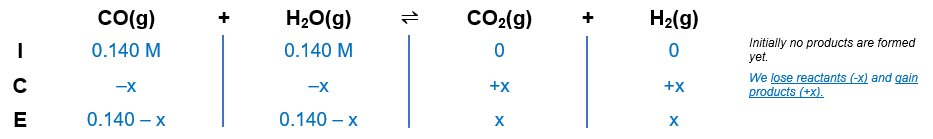# Problem: Consider the reaction CO(g) + H2O(g) ⇌ CO2(g) + H2(g); Kc = 102 at 500 KA reaction mixture initially contains 0.140 M CO and 0.140 M H2O. What will be the equilibrium concentration of H2O?

###### FREE Expert Solution

CO(g) + H2O(g) ⇌ CO2(g) + H2(g); Kc = 102 at 500 K

Step 1: Construct an ICE chartStep 2: Calculate the change (x) in the reaction using the equilibrium constant.

85% (443 ratings)###### Problem Details
Consider the reaction CO(g) + H2O(g) ⇌ CO2(g) + H2(g); Kc = 102 at 500 K
A reaction mixture initially contains 0.140 M CO and 0.140 M H2O. What will be the equilibrium concentration of H2O?